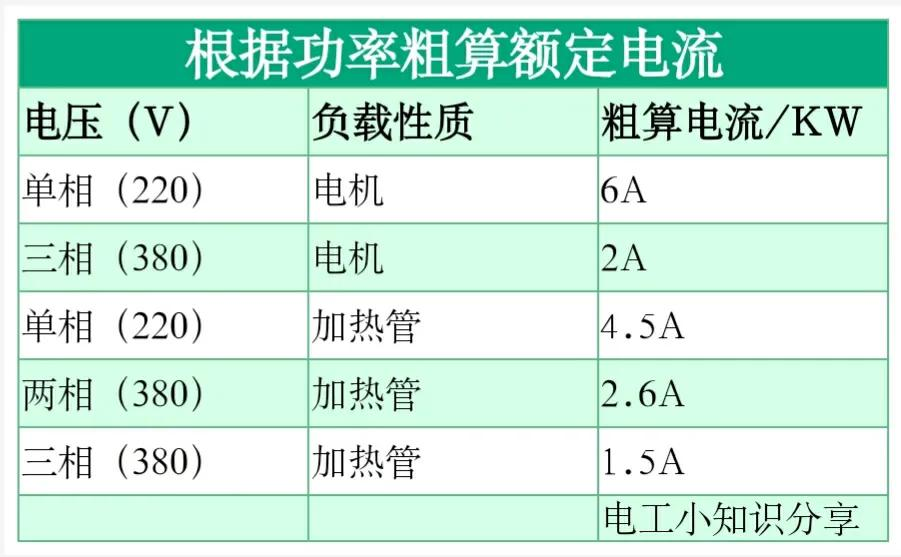# 1KW三相电机电流

P=3UICOS

I=P3uCOS

=10001.7323800.850.9=1.982A

# 1KW单相电机电流

P=UICOS

I=p \u \cos

=1000 \220 \0.85 \0.9=5.946A

0.75KW电机：0.75 2=1.5a

1.1千瓦电机：1.12=2.2A

0.75KW电机：0.75 6=4.5a

1.1KW电机：1.1 6=6.6a。

# 三相电压功率1KW加热管的额定电流

P=3UI

I=P3U

=1000 1.732 380=1.5A

P=UI

I=p \u

=1000380=2.6A

# 单相电压功率1KW加热管的额定电流

P=UI

I=p \u

=1000220=4.5A### 在线留言选型？样本？参数？采购？请第一时间反馈给我们石头制砂机多少钱一套？

石头制砂机多少钱一套？

石头制砂机多少钱一套？

防爆电机大概有哪些？

ABB电机系列有哪些？

ABB电机系列有哪些？

• #### 李总

ABB电机型号大全及含义是哪些？

石头制砂机多少钱一套？

ABB电机系列有哪些？